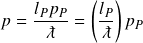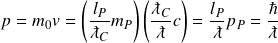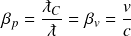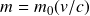# Wavelength operator

The wavelength operator appears frequently in quantum mechanics because it specifies a particle’s wavelength. The operator is a value between 0 and 1 representing the inverse number of natural length units in the wavelength. Dividing 1 by the wavelength operator gives the wavelength in natural units, and dividing the Planck length by the operator gives the wavelength in SI units.

The wavelength operator is a spatial operator applied in each of the three unit dimensions. It describes two important attributes of a particle in the length and mass unit dimensions. The length dimension gives the particle’s wavelength, while applying the operator in the mass dimension provides a measure of the particle’s energy potential that is inversely proportional to the wavelength. The operator applies to particles with and without rest mass.

Applying the wavelength operator in the time dimension does not yield a physical attribute of the particle, but is included by historical convention as described below.

The New Foundations Model shows the wavelength operator as a single line across the three unit dimensions. The potential in each unit dimension is reduced proportionally.

The three correlated unit dimensions give three equivalent forms of the wavelength operator–one in each unit dimension:

The correlation between length and mass potential appears in a number of equations that find wavelength from mass/momentum and mass/momentum from wavelength–including the Compton wavelength and de Broglie wavelength formulas.

The wavelength operator quantifies Part I of the 2-part energy mechanism–the energy potential of a particle’s spatial distribution. The first part of the mechanism is historically denoted in unit dimensions of momentum, LMT-1, with a maximum potential of 𝑙𝑃𝑚𝑃 / 𝑡𝑃.

Applying the wavelength operator to the momentum potential produces observables of wavelength and inertial mass. The proportional quantity of time, denoted 𝑇𝜆, represents wavelength in the time dimension.

### Observable

While only one instance of the operator is applied in formulas, it is easy to see that a single application is equivalent to applying the operator in each of the three dimensions LMT-1.

## Wavelength operator in historical formulas

The wavelength operator is hidden by the composite structure of Planck’s constant in historical formulas, where the ratio of Planck’s constant to a particle’s wavelength creates the operator. For example, the momentum formulacan be restated in natural units by replacing ℏ with 𝑙𝑃𝑚𝑃𝑐, or simply 𝑙𝑃𝑝𝑃The wavelength operator is formed from the ratio of Planck length (hidden in the constant) to the particle’s wavelength. In this formula, the operator reduces the maximum momentum potential in the right proportion to produce the particle’s momentum.

## The physical meaning of momentum

Momentum is historically defined for particles that have rest mass as the product of rest mass and velocity. But this classical representation is incompatible with the definition of momentum used for elementary particles that have no rest mass. In photons, for example, momentum is determined entirely by a particle’s wavelength and not its velocity.

A consistent definition of momentum arises for all particles when we treat momentum as the potential due strictly to a particle’s wavelength and not its velocity. Each of these two factors–wavelength and velocity– contribute to a particle’s kinetic energy, but only wavelength gives consistent meaning to momentum for particles with and without rest mass. Momentum is analogous to a payload quantified by the concentration of a particle’s wavelength, while velocity delivers the payload as energy.

The misleading formula 𝑝 = 𝑚0𝑣 only produces a meaningful quantity of potential because the ratio of velocity to the speed of light (𝑣/𝑐) is equal to the ratio of Compton wavelength to de Broglie wavelength ( ƛ𝐶 /ƛ). Rest mass quantifies the Compton wavelength, and velocity transforms it into the de Broglie wavelength.

The concept of momentum pre-dates quantum theory when wave-like attributes of matter first became known. For Newton and others who described momentum prior to the 20th century, the quantity 𝑣2 simply matched the observational data. Multiplying rest mass by velocity squared correctly modeled the relationship between rest mass and energy, but it required additional unit dimensions of LT-1. These additional unit dimensions resolve the mathematical proportionality at the expense of an accurate representation of the particle’s physical state. A dimensionless transformation of the particle’s wavelength from Compton wavelength to de Broglie wavelength is what was called for, but wavelength was an unknown and unobservable attribute at the time.

Louis de Broglie’s introduction of the quantum mechanical formula for momentum clarifies the separate roles played by wavelength and velocity in determining kinetic energy. The elementary form of de Broglie’s equation shows how the classical and quantum mechanical formulas are relatedThere is no physical explanation for squaring a particle’s velocity to produce kinetic energy, but there is a simple and comprehensive explanation for kinetic energy as the product of wavelength potential and velocity.

The physical meaning of momentum and kinetic energy is restored when we replace the classical definition of momentumwith the natural formula.

The concept of momentum in dimensions LMT-1 is replaced by inertial mass in dimension M which is effective for all particles including photons and electrons. Velocity is strictly a representation of a particle’s rate of displacement, and energy is the product of these two factors in dimensions LMT-1.

It is pragmatic to use rest mass and velocity to calculate inertial mass and kinetic energy. Especially in many-particle systems, velocity is much easier to measure than the average wavelength of the particles. But velocity should be understood as a means for calculating wavelength and not as a literal description of the particle’s inertial mass/momentum potential.

In the language of The New Foundations Model, the velocity operator is equal to the momentum operator as shown in the following relational diagram of the mechanical operators:

The relationship between the velocity and momentum operators can also be expressed as## Momentum in the New Foundations Model

The New Foundations Model explains physics in terms of the preferred concept of inertial mass, but it also reconciles traditional unit dimensions of momentum and energy. This is accomplished by distinguishing inertial mass 𝑚, which represents the inertial strength of matter and energy, from 𝑚0 which represents the latent form of rest mass..

Multiplying inertial mass by 𝑐 is equivalent to multiplying rest mass by 𝑣 in traditional unit dimensions of momentum and energy. The time dimension of momentum has no physical meaning so the model uses 𝑇𝜆 to convey the meaning of wavelength. The ratio ƛ / 𝑇𝜆 is always equal to the speed of light to translate from inertial mass into traditional units of momentum.

The following illustration shows the equivalence between New Foundations Model momentum and traditional momentum.

=

The beauty and elegance of this approach is demonstrated in the table of photon mechanics, where the mechanical attributes of a photons are described simply by applying the wavelength operator in each unit dimension.

Wavelength operator